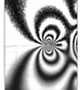Lecture Notes 1. Introduction 2. Tensors 3. Hookes Law 4. Fundamental Equations 5. Elastic Rod 6. 2D Elasticity 10. Contact 13. Wedge 15. Dislocations

ME 340A Theory and Applications of ElasticityThis course provides an introduction to the elasticity theory and its application to material structures at microscale. The basic theory includes the definition of stress, strain and elastic energy; equilibrium and compatibility conditions; and the formulation of boundary value problems. We will discuss two major methods for solving elasticity equations: the stress function method for 2D problems and the Green’s function approach for 3D problems. The theory and solution methods are then applied to microscopic defects in solids, their stress fields and interaction with each other. Analytic and numerical tools will be developed to solve elasticity problems.  Textbook: J. R. Barber, Elasticity, 2nd Edition. Kluwer Academic Publishers (2002).

All Notes in One PDF File